Big factorial

How many zeros end number 116! ?

Result

n =  27

Solution:

116! = 3 3931086844 5189820119 8256093588 5732032396 6355569942 0770196366 2088123265 3141763303 3625453597 1207181169 6988685849 9194160778 0111073928 2362611996 0469179757 0505851011 0720000000 0000000000 0000000000

Leave us a comment of this math problem and its solution (i.e. if it is still somewhat unclear...):Be the first to comment!Next similar math problems:

1. Committees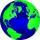How many different committees of 6 people can be formed from a class of 30 students?
2. Cinema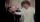How many ways can be divided 11 free tickets to the premiere of "Jáchyme throw it in the machine" between 6 pensioners?
3. Colors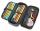Willie got birthday 6 colour pens in different colors. How many ways he can give them side by side in pencil?
4. WordsHow many 3 letter "words" are possible using 14 letters of the alphabet? a) n - without repetition b) m - with repetition
5. Permutations without repetitionFrom how many elements we can create 720 permutations without repetition?
6. Cards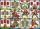How many ways can give away 32 playing cards to 5 player?
7. Value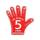Find the value of the expression: 6!·10^-3
8. Fish tankA fish tank at a pet store has 8 zebra fish. In how many different ways can George choose 2 zebra fish to buy?
9. CandiesIn the box are 12 candies that look the same. Three of them are filled with nougat, five by nuts, four by cream. At least how many candies must Ivan choose to satisfy itself that the selection of two with the same filling? ?
10. Theorem proveWe want to prove the sentence: If the natural number n is divisible by six, then n is divisible by three. From what assumption we started?
11. Median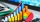The number of missed hours was recorded in 11 pupils: 5,12,6,8,10,7,5,110,2,5,6. Determine the median.
12. Virus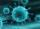We have a virus that lives one hour. Every half hour produce two child viruses. What will be the living population of the virus after 3.5 hours?
13. CardsSuppose that are three cards in the hats. One is red on both sides, one of which is black on both sides, and a third one side red and the second black. We are pulled out of a hat randomly one card and we see that one side of it is red. What is the probabi
14. 75th percentile (quartille Q3)Find 75th percentile for 30,42,42,46,46,46,50,50,54
15. EquationEquation ? has one root x1 = 8. Determine the coefficient b and the second root x2.
16. Six termsFind the first six terms of the sequence a1 = -3, an = 2 * an-1
17. Geometric progression 2There is geometric sequence with a1=5.7 and quotient q=-2.5. Calculate a17.